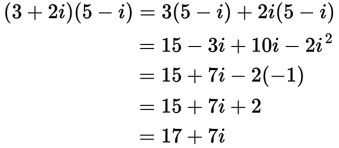# SAT Math Multiple Choice Question 314: Answer and Explanation

### Test Information

Question: 314

14. If a + bi represents the complex number that results from multiplying 3 + 2i times 5 - i, what is the value of a?

• A. 2
• B. 13
• C. 15
• D. 17

Explanation:

D

Difficulty: Medium

Category: Additional Topics in Math / Imaginary Numbers

Strategic Advice: Multiply the two complex numbers just as you would two binomials (using FOIL). Then, combine like terms and use the definition i2 = -1 to simplify the result.